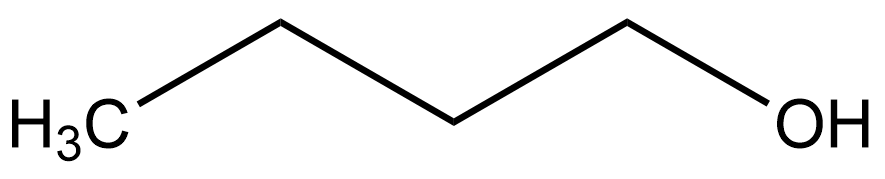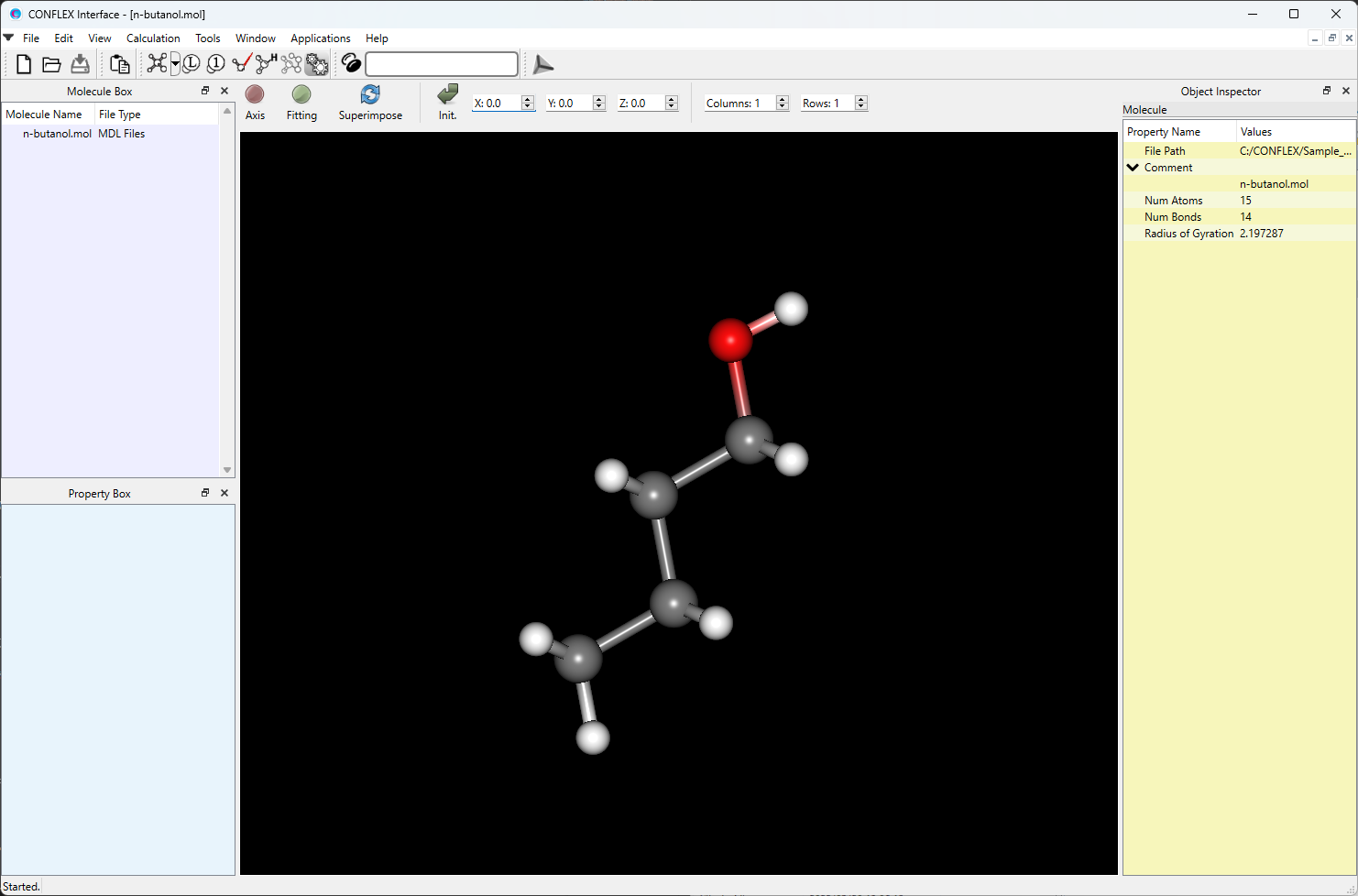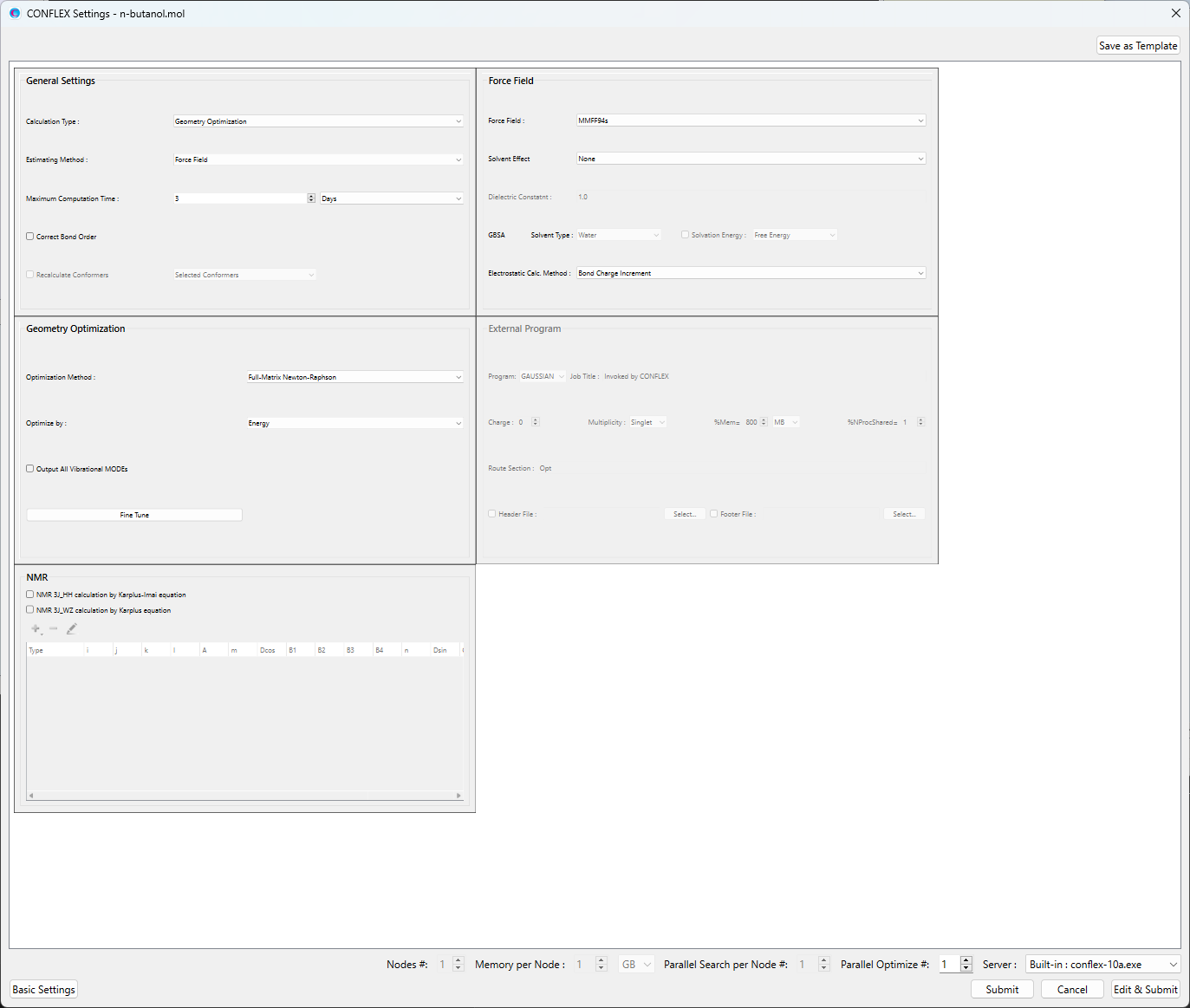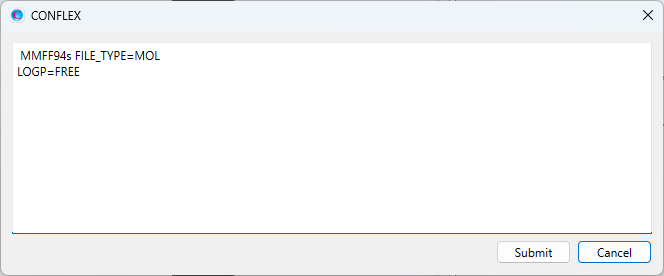# Calculation of water/octanol partition coefficient

## [Calculation of log P value]

On a molecular design of drug materials, one of the important characters for estimating a molecule designed is partition coefficient log P. The log P is common logarithm of a ratio P of concentrations of a solute between two solvents like water and organic solvents and is frequently used as the important character to estimate hydrophilicity and hydrophobicity of the solute.

We can calculate the log P value from solvation free energies of the solute in the water and organic solvents.
CONFLEX can automatically calculate the water/octanol partition coefficient log Pow value by calculating the solvation free energies in the water and octanol solvents based on GB/SA model. About calculation of solvation free energy based on GB/SA model, please refer to [Calculation of solvation free energy].

## [Relationship of log P value and solvation free energy]

The water/octanol partition coefficient log Pow value and the solvation free energies (ΔGwat, ΔGoct) in the water and octanol solvents are related bywhere the R is gas constant, and the T is absolute temperature.

## [Calculation of log P value]

This section explains how to calculate the log Pow of 1-Butanol as an example.Structure data of 1-Butanol (1-butanol.mol)

```1-butanol.mol

15 14  0  0  0  0  0  0  0  0999 V2000
-1.1802   -1.8691   -0.0000 C   0  0  0  0  0  0  0  0  0  0  0  0
0.1388   -1.1076   -0.0000 C   0  0  0  0  0  0  0  0  0  0  0  0
-0.1388    0.3899   -0.0000 C   0  0  0  0  0  0  0  0  0  0  0  0
1.1802    1.1514   -0.0000 C   0  0  0  0  0  0  0  0  0  0  0  0
0.9247    2.5299   -0.0000 O   0  0  0  0  0  0  0  0  0  0  0  0
-0.9774   -2.9634   -0.0000 H   0  0  0  0  0  0  0  0  0  0  0  0
-1.7631   -1.6004    0.9093 H   0  0  0  0  0  0  0  0  0  0  0  0
-1.7637   -1.6001   -0.9088 H   0  0  0  0  0  0  0  0  0  0  0  0
0.7217   -1.3763   -0.9093 H   0  0  0  0  0  0  0  0  0  0  0  0
0.7223   -1.3766    0.9088 H   0  0  0  0  0  0  0  0  0  0  0  0
-0.7217    0.6586    0.9093 H   0  0  0  0  0  0  0  0  0  0  0  0
-0.7223    0.6589   -0.9088 H   0  0  0  0  0  0  0  0  0  0  0  0
1.7631    0.8827   -0.9093 H   0  0  0  0  0  0  0  0  0  0  0  0
1.7637    0.8824    0.9088 H   0  0  0  0  0  0  0  0  0  0  0  0
1.7610    2.9634   -0.0000 H   0  0  0  0  0  0  0  0  0  0  0  0
1  2  1  0
1  6  1  0
1  7  1  0
1  8  1  0
2  3  1  0
2  9  1  0
2 10  1  0
3  4  1  0
3 11  1  0
3 12  1  0
4  5  1  0
4 13  1  0
4 14  1  0
5 15  1  0
M  END```

### [Execution by Interface]

Open the 1-butanol.mol file by CONFLEX Interface.Select [CONFLEX] in Calculation menu, and click in the calculation setting dialog displayed. A detail setting dialog will be displayed.Click at bottom right in the detail setting dialog, and add [LOGP=FREE] to the dialog displayed. By adding the keyword, the log Pow will be calculated by calculating the solvation free energies based on vibrational analyses in the water and octanol solvents.When the calculation settings are complete, click . The calculation will start.

[LOGP=] keyword has three options of [FREE], [OPTIMZ], and [SINGLE]. By changing the option, you can select an approximate level for calculating the solvation free energies. About the correspondence between each option and the approximation level, please refer to [Calculation of solvation free energy].

### [Execution by command line]

The calculation settings are defined by describing keywords in the 1-butanol.ini file.

1-butanol.ini file

`MMFF94S LOGP=FREE`

[LOGP=FREE] means that the log Pow will be calculated by calculating the solvation free energies based on vibrational analyses in the water and octanol solvents.
[MMFF94S] means that MMFF94s force field is used.

[LOGP=] keyword has three options of [FREE], [OPTIMZ], and [SINGLE]. By changing the option, you can select an approximate level for calculating the solvation free energies. About the correspondence between each option and the approximation level, please refer to [Calculation of solvation free energy].

Store the 1-butanol.mol and 1-butanol.ini files in an one folder, and execute below command. The calculation will start.

`C:\CONFLEX\bin\flex9a_win_x64.exe   -par   C:\CONFLEX\par   1-butanolenter`

The above command is for Windows OS. For the other OS, please refer to [How to execute CONFLEX].

## Calculation results

Results of the log Pow calculation for 1-Butanol are shown below (1-butanol.bso). The experimental value of log Pow is 0.88.
The calculations are performed in order of gas phase, octanol solvent, and water. When the calculations in the octanol and water solvents finished, each solvation free energy will be shown.

Solvation free energy of 1-Butanol in the octanol solvent

```SOLVATION ENERGY (DIFF. OF TOTAL ENERGY) =  -5.85364 (KCAL/MOL)

SOLVATION ENERGY (DIFF. OF  FREE ENERGY) =  -5.92111 (KCAL/MOL)```

Solvation free energy of 1-Butanol in the water

```SOLVATION ENERGY (DIFF. OF TOTAL ENERGY) IN WATER =  -4.50971 (KCAL/MOL)

SOLVATION ENERGY (DIFF. OF  FREE ENERGY) IN WATER =  -4.72882 (KCAL/MOL)```

Whe all calculations finished, the log Pow value, 0.875, calculated by the solvation free energies is outputted at the end of bso file.

Summary of the log Pow calculation (1-butanol.bso file)

``` !------------------------------------------------------------------------------------!
!                                                                                    !
!                        *** SUMMARY OF LOGP CALCULATION ***                         !
!                                                                                    !
!            FORMULA: LOGP = (DELTAG(WATER) - DELTAG(OCTANOL))/(2.30*R*T)            !
!                     (R = 1.9872065 (CAL/(K*MOL))  T = 298.15 (KELVIN))             !
!                                                                                    !
!                     DELTAG(WATER)   =  -4.72882 (KCAL/MOL)                         !
!                     DELTAG(OCTANOL) =  -5.92111 (KCAL/MOL)                         !
!                                                                                    !
!                                LOGP =  0.875                                       !
!                                                                                    !
!------------------------------------------------------------------------------------! ```